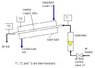# Heat Transfer

dj_silver
Thought this would be the most appropriate forum to ask for help

Air flows through a copper inside tube and is heated by saturated steam condensing on the outside of the tube.

1. Determine the rate of heat transfer to the air at 3 different flow rates of the air (rotameter readings 10, 20 and 30).
For each air flow rate:
2. Experimentally determine the overall heat transfer coefficient based on the outside area of the pipe.
3. Estimate the inside (air) film heat transfer coefficient assuming that the resistances of the copper wall and steam film are small compared to the resistance of the air film.
4. Predict the film heat transfer coefficient using the Dittus-Boelter equations.
5. Compare the film heat transfer coefficients from the experiments with those calculated from the Dittus-Boelter equation. Comment on the comparison.
6. Estimate the steam consumption, stating assumptions.

The schematic is attached.

The dimensions of central copper tube are:
Length: L = 300 mm
Inside diameter: di = 4.5 mm
Outside diameter: do = 6.3 mm

Just wondering what the best way to approach this is, and how to answer all the questions, what measurements to make etc?

Thanks very much

#### Attachments

•schematic.JPG
16.4 KB · Views: 627